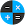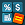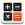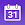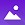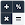# Simple Calculator: Quick math

by Simple Mobile Tools
100,000+ installs

Android application Simple Calculator: Quick math developed by is listed under category Tools. According to Google Play Simple Calculator: Quick math achieved more than 100,000 installs. Simple Calculator: Quick math currently has 1,235 ratings with average rating value of 4.23. The current percentage of ratings achieved in last 30 days is 2.7%, percentage of ratings achieved in last 60 days is 5.69%. Simple Calculator: Quick math has the current market position #114584 by number of ratings. A sample of the market history data for Simple Calculator: Quick math can be found below. Last update on 2022-05-18.

Title: Simple Calculator: Quick math Simple Mobile Tools Tools Free Android
Total ratings: 1235 2.7% 5.69% 4.23
Installs (achieved): 100,000+ 173,455
 5 star ratings: 860 117 110 7 117

2021-04-02: Android application Simple Calculator: Quick math achieved 100,000 installs. Android application Simple Calculator: Quick math achieved 50,000 installs. Android application Simple Calculator: Quick math achieved 10,000 installs.

Total number of ratings
Total number of active users rated for Simple Calculator: Quick math.

Total number of installs (*estimated)
Estimation of total number of installs on Google Play. Approximated from number of ratings and install bounds achieved on Google Play.

Average rating
Average rating value on Google Play. Given by active users of the application.